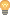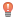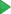# Tutorial. Replace conditional logic with strategy pattern

When you have a method with lots of conditional logic (i.e., if statements), you're asking for trouble. Conditional logic is notoriously difficult to manage, and may cause you to create an entire state machine inside a single method.

## Analyzing the sample application

Here's a short example. Let's say, there is a method that calculates insurance costs based on a person's income:

```package ifs; public class IfElseDemo { public double calculateInsurance(double income) { if (income <= 10000) { return income*0.365; } else if (income <= 30000) { return (income-10000)*0.2+35600; } else if (income <= 60000) { return (income-30000)*0.1+76500; } else { return (income-60000)*0.02+105600; } } }```

Let's analyze this example. Here we see the four "income bands widths", separated into four calculation strategies. In general, they conform to the following formula:

`(income - adjustment) * weight + constant`

Our goal is to provide separate classes to calculate each strategy, and transform the original class to make it more transparent.

## Creating and running test

First of all, let's make sure that the class works. To do that, create a test class, using the JUnit4 testing framework.

Place the caret at the class name, and then press Alt+Enter (or click). From the list of suggested intention actions, choose Create Test:

In the Create Test dialog, choose JUnit4 from the Testing library drop-down list, click Fix, if you do not have JUnit library, and then click OK:

The stub test class is created. However, you have to provide some meaningful code, using the provided quick fix () to create import statement:

```import org.junit.Test; import static org.junit.Assert.*; public class IfElseDemoTest { @Test public void low() { assertEquals(1825, insuranceFor(5000), 0.01); } @Test public void medium() { assertEquals(38600, insuranceFor(25000), 0.01); } @Test public void high() { assertEquals(78500, insuranceFor(50000), 0.01); } @Test public void veryHigh() { assertEquals(106400, insuranceFor(100_000), 0.01); } private double insuranceFor(double income) { return new IfElseDemo().calculateInsurance(income); } }```

Now let's run this test by clicking the run buttonin the left gutter, or by pressing Ctrl+Shift+F10:

All 4 tests pass:

## Extracting methods

Next, bring forward the original class, place the caret at the expression

`(income-60000)*0.02+105600`

and invoke Extract Method Dialog dialog (Ctrl+Alt+M):

You get the following code:

```package ifs; class IfElseDemo { public double calculateInsurance(double income) { if (income <= 10000) { return income*0.365; } else if (income <= 30000) { return (income-10000)*0.2+35600; } else if (income <= 60000) { return (income-30000)*0.1+76500; } else { return calculateInsuranceVeryHigh(income); } } public double calculateInsuranceVeryHigh(double income) { return (income-60000)*0.02+105600; } }```

Next, use the same Extract Method refactoring for for 60000, 0.02 and 105600 fragments, and create the methods `getAdjustment`, `getWeight` and `getConstant`:

```package ifs; class IfElseDemo { public double calculateInsurance(double income) { if (income <= 10000) { return income*0.365; } else if (income <= 30000) { return (income-10000)*0.2+35600; } else if (income <= 60000) { return (income-30000)*0.1+76500; } else { return calculateInsuranceVeryHigh(income); } public double calculateInsuranceVeryHigh(double income) { return (income- getAdjustment())* getWeight() + getConstant(); } public int getConstant() { return 105600; } public double getWeight() { return 0.02; } public int getAdjustment() { return 60000; } }```

## Using Extract Delegate refactoring

Next, select all the methods created in the previous chapter, and invoke the Extract Delegate refactoring:

The newly created class has the name `InsuranceStrategyVeryHigh`.

Then delete all the selected methods from the class `IfElseDemo`. Thus you get the following two classes:

```package ifs; class IfElseDemo { private final InsuranceStrategyVeryHigh insuranceStrategyVeryHigh = new InsuranceStrategyVeryHigh(); public double calculateInsurance(double income) { if (income <= 10000) { return income*0.365; } else if (income <= 30000) { return (income-10000)*0.2+35600; } else if (income <= 60000) { return (income-30000)*0.1+76500; } else { return insuranceStrategyVeryHigh.calculateInsuranceVeryHigh(income); } }```

and

```package ifs; public class InsuranceStrategyVeryHigh { public InsuranceStrategyVeryHigh() { } public double calculateInsuranceVeryHigh(double income) { return (income - getAdjustment()) * getWeight() + getConstant(); } public int getConstant() { return 105600; } public double getWeight() { return 0.02; } public int getAdjustment() { return 60000; } }```

## Fine tuning

This code requires some modifications. First, let's change the class `IfElseDemo`:

• Rename (Shift+F6) the field `insuranceStrategyVeryHigh` to `strategy`
• Make this field not final.
• Using the intention action, split it into declaration and initialization:
• Move the initialization down to the corresponding `if-else` branch.

Get the following code:

```package ifs; class IfElseDemo { private InsuranceStrategyVeryHigh strategy; public double calculateInsurance(double income) { if (income <= 10000) { return income*0.365; } else if (income <= 30000) { return (income-10000)*0.2+35600; } else if (income <= 60000) { return (income-30000)*0.1+76500; } else { strategy = new InsuranceStrategyVeryHigh(); return strategy.calculateInsuranceVeryHigh(income); } } }```

Next, let's modify the class `InsuranceStrategyVeryHigh` - invoke the Extract Superclass refactoring for it.

Mind the settings in the Extract Superclass Dialog dialog:

• The name of the superclass to be generated is `InsuranceStrategy`.
• All the methods of the class `InsuranceStrategyVeryHigh` are checked - it means that they will be included in the superclass.
• The method `calculateInsuranceStrategyVeryHigh` remains non-abstract; all the other methods are made abstract by selecting the Make Abstract check boxes.

Agree to replace the usage of `InsuranceStrategyVeryHigh` class (in `IfElseDemo` class) with the superclass, and get the following `InsuranceStrategy` class:

```package ifs; public abstract class InsuranceStrategy { public double calculateInsuranceVeryHigh(double income) { return (income - getAdjustment()) * getWeight() + getConstant(); } public abstract int getConstant(); public abstract double getWeight(); public abstract int getAdjustment(); }```

## Implementing the abstract class

Next, for this abstract class, use Implement Abstract Class intention to create implementations for all strategies:

Name the new implementation classes `InsuranceStrategyLow`, `InsuranceStrategyMedium` and `InsuranceStrategyHigh`.

For all new implementations provide correct `return` statements for the methods `getAdjustment()`, `getWeight()` and `getConstant()`.

Thus all implementation classes should look similar to the class `InsuranceStrategyVeryHigh`, but with strategy-specific adjustment, weight and constant. For example:

```package ifs; public class InsuranceStrategyMedium extends InsuranceStrategy { @Override public int getConstant() { return 35600; } @Override public double getWeight() { return 0.2; } @Override public int getAdjustment() { return 10000; } }```

Note that in all newly created implementation classes the class names are grey - they are not used so far.

Next, bring forward the class `IfElseDemo`, and modify all the branch bodies so that they initialize the `strategy` field, like in the last branch:

```package ifs; class IfElseDemo { private InsuranceStrategy strategy; public double calculateInsurance(double income) { if (income <= 10000) { strategy = new InsuranceStrategyLow(); return strategy.calculateInsuranceVeryHigh(income); } else if (income <= 30000) { strategy = new InsuranceStrategyMedium(); return strategy.calculateInsuranceVeryHigh(income); } else if (income <= 60000) { strategy = new InsuranceStrategyHigh(); return strategy.calculateInsuranceVeryHigh(income); } else { strategy = new InsuranceStrategyVeryHigh(); return strategy.calculateInsuranceVeryHigh(income); } } }```

Finally, rename the method `calculateInsuranceVeryHigh`: bring forward the class `InsuranceStrategy`, place the caret at the method name and press Shift+F6. The new name should be `calculate`.

## Happy end

And finally enjoy the code:

```package ifs; class IfElseDemo { private InsuranceStrategy strategy; public double calculateInsurance(double income) { if (income <= 10000) { strategy = new InsuranceStrategyLow(); return strategy.calculate(income); } else if (income <= 30000) { strategy = new InsuranceStrategyMedium(); return strategy.calculate(income); } else if (income <= 60000) { strategy = new InsuranceStrategyHigh(); return strategy.calculate(income); } else { strategy = new InsuranceStrategyVeryHigh(); return strategy.calculate(income); } } }```

Next, let's run the test class again. All tests should pass – we have refactored the code, but it still produces the same results.